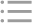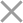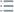To get a trial key
fill out the form below
** By clicking this button you agree to our Privacy Policy statementRequest our prices
--Select currency--
USD
EUR
RUB
* By clicking this button you agree to our Privacy Policy statementFree PVS-Studio license for Microsoft MVP specialists
** By clicking this button you agree to our Privacy Policy statementTo get the licence for your open-source project, please fill out this form
** By clicking this button you agree to our Privacy Policy statementI am interested to try it on the platforms:
 Windows Linux macOS PVS-Studio for .NET Core JetBrains Rider
** By clicking this button you agree to our Privacy Policy statementMessage submitted.

Your message has been sent. We will email you at

If you haven't received our response, please do the following:
check your Spam/Junk folder and click the "Not Spam" button for our message.
This way, you won't miss messages from our team in the future.

>
>
>
V695. Range intersections are possible …Introduction
Analyzing projects
On Windows
On Linux and macOS
Continuous use of the analyzer in software development
Deploying the analyzer in cloud Continuous Integration services
Managing analysis results
Additional configuration and resolving issues
Analyzer diagnostics
General Analysis (C++)
General Analysis (C#)
General Analysis (Java)
Diagnosis of micro-optimizations (C++)
Diagnosis of 64-bit errors (Viva64, C++)
Customer specific requests (C++)
MISRA errors
AUTOSAR errors
OWASP errors (C++)
OWASP errors (C#)
OWASP errors (Java)
Problems related to code analyzerContents

# V695. Range intersections are possible within conditional expressions.

May 29 2014

The analyzer has detected a potential error in a condition. The program must perform different actions depending on which range of values a certain variable meets.

For this purpose, the following construct is used in the code:

``````if ( MIN_A < X && X < MAX_A ) {
....
} else if ( MIN_B < X && X < MAX_B ) {
....
}``````

The analyzer generates the warning when the ranges checked in conditions overlap. For example:

``````if ( 0 <= X && X < 10)
FooA();
else if ( 10 <= X && X < 20)
FooB();
else if ( 20 <= X && X < 300)
FooC();
else if ( 30 <= X && X < 40)
FooD();``````

The code contains a typo. The programmer's fingers faltered at some moment and he wrote "20 <= X && X < 300" instead of "20 <= X && X < 30" by mistake. If the X variable stores, for example, the value 35, it will be the function FooC() that will be called instead of FooD().

The fixed code:

``````if ( 0 <= X && X < 10)
FooA();
else if ( 10 <= X && X < 20)
FooB();
else if ( 20 <= X && X < 30)
FooC();
else if ( 30 <= X && X < 40)
FooD();``````

Here is another example:

``````const int nv_ab = 5;
const int nv_bc = 10;
const int nv_re = 15;
const int nv_we = 20;
const int nv_tw = 25;
const int nv_ww = 30;
....
if (n < nv_ab) { AB(); }
else if (n < nv_bc) { BC(); }
else if (n < nv_re) { RE(); }
else if (n < nv_tw) { TW(); }   // <=
else if (n < nv_we) { WE(); }   // <=
else if (n < nv_ww) { WW(); }``````

Depending on the value of the 'n' variable, different actions are performed. Poor variable naming may confuse a programmer - and so it did in this example. The 'n' variable should have been compared to 'nv_we' first and only then to 'nv_tw'.

To make the mistake clear, let's substitute the values of the constants into the code:

``````if (n < 5) { AB(); }
else if (n < 10) { BC(); }
else if (n < 15) { RE(); }
else if (n < 25) { TW(); }
else if (n < 20) { WE(); } // Condition is always false
else if (n < 30) { WW(); }``````

The fixed code:

``````if (n < nv_ab) { AB(); }
else if (n < nv_bc) { BC(); }
else if (n < nv_re) { RE(); }
else if (n < nv_we) { WE(); }    // <=
else if (n < nv_tw) { TW(); }    // <=
else if (n < nv_ww) { WW(); }``````

This diagnostic is classified as:

 CWE-561CWE-670CERT-MSC01-C
 You can look at examples of errors detected by the V695 diagnostic.Our website uses cookies to enhance your browsing experience. Would you like to learn more?